﻿ 广义极值分布参数估计方法比较研究 Comparative Study on Parameter Estimation Methods of Generalized Extreme Value Distribution

Journal of Water Resources Research
Vol.05 No.03(2016), Article ID:17749,9 pages
10.12677/JWRR.2016.53033

Comparative Study on Parameter Estimation Methods of Generalized Extreme Value Distribution

Ling Xiao, Shuangchao Lei

Zunyi Survey and Design Institute of Water Conservancy and Hydropower, Zunyi Guizhou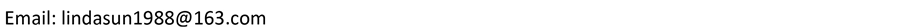Received: May 16th, 2016; accepted: Jun. 3rd, 2016; published: Jun. 6th, 2016ABSTRACT

Research on parameter estimation of Generalized Extreme Value distribution based on the moment, the probability weighted moment and the higher probability weighted moments. The two examples of Wujia Yuanzi and Jiang Bin stations annual maximum flow series were analyzed by the three parameter estimation methods of GEV distribution, which included the moment estimation, the probability weighted moment estimation and the higher probability weighted moment estimation. The results indicate that using the higher PWMs to fit the large flood values are much better than the moment and the probability weighted moment, and we can use it to estimate the parameters of the flood frequency distribution. The Monte Carlo experiments indicate that the SE, Bias and RMSE in the design floods of different return periods which based on the higher probability weighted moments are smaller and the PWMs have higher accuracy than the moment and the probability weighted moment.

Keywords:Generalized Extreme Value Distribution, Higher Probability Weighted Moments, Method of Moment, Parameter Estimation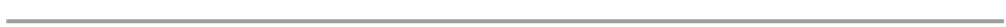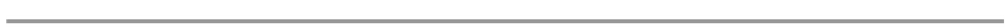1. 引言

2. 广义极值分布(1)(2)(3)

3. 参数估计方法

3.1. 高阶概率权重矩法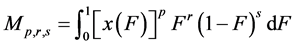(4)(5)(6)(7)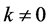时，Wang Q.J.  推求了GEV分布的概率权重矩为：(8)(9)(10)(11)(12)(13)(14)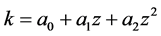(15)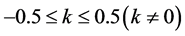，分别给定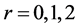(即普通概率权重矩)、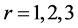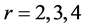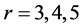，利用式(14)求出不同阶数对应的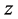值，并对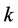的关系进行拟合，得出不同阶数下式(15)中的系数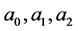，结果见表1(16)(17)

3.2. 普通矩法(18)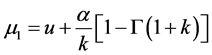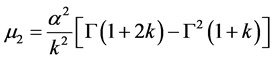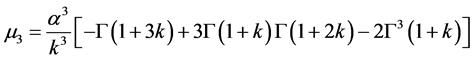(19)，利用式(19)求出不同对应的值，并对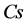的关系进行拟合(结果见图1)，得出其关系式，并通过其关系式求的初值，再利用牛顿迭代法计算出满足条件的值。将求出的值带入式(20)、(21)即可求解参数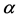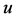(20)(21)

4. 实例应用Table 1. Coefficients a0, a1 and a2 and the absolute error for different higher probability weighted moments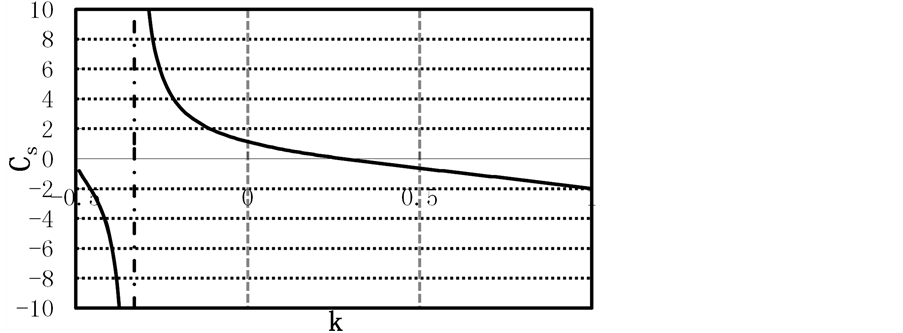Figure 1. Relationship between the Cs and k for the GEV distribution

5. Monte Carlo试验Table 2. The estimated GEV distribution parameters of several methods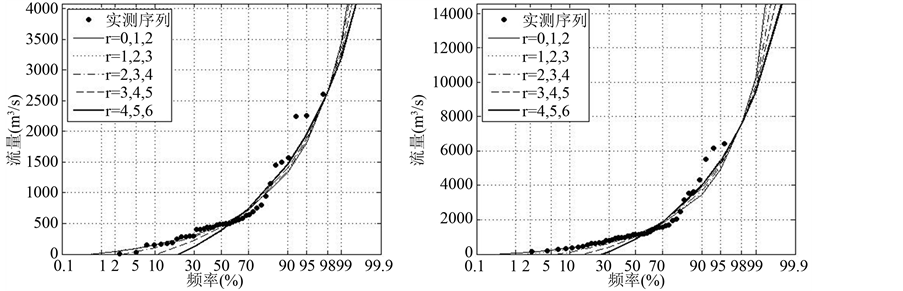(I) 五家院子 (II) 江滨

Figure 2. Fitting of the GEV distribution using different higher PWMs to annual maximum flows at different stations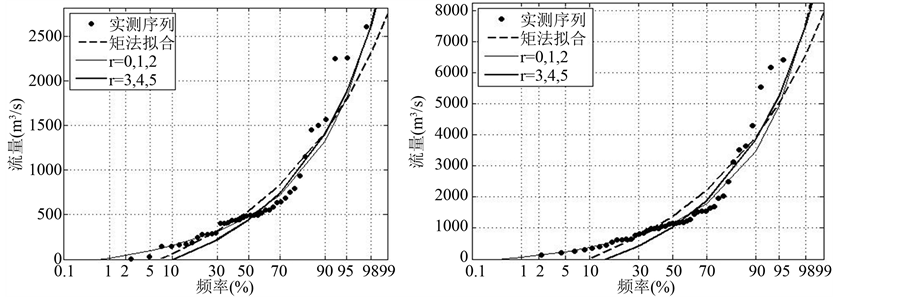(I) 五家院子 (II) 江滨

Figure 3. Fitting of the GEV distribution using different methods to annual maximum flows at different stationsTable 3. SE, Bias and RMSE in x(F=0.98) estimates from fitting the GEV distribution with Wakeby samplesTable 4. SE, Bias and RMSE in x(F = 0.99)estimates from fitting the GEV distribution with Wakeby samples

6. 结论

Comparative Study on Parameter Estimation Methods of Generalized Extreme Value Distribution[J]. 水资源研究, 2016, 05(03): 262-270. http://dx.doi.org/10.12677/JWRR.2016.53033

1. 1. 陈子燊, 刘曾美, 陆剑飞. 广义极值分布参数估计方法的对比研究[J]. 中山大学学报(自然科学版), 2010, 49(6): 105-109. CHEN Zishen, LIU Zengmei, LU Jianfei. Comparative analysis of parameter estimation methods of general extreme value dis-tribution. Acta Scientiarum Naturalium Universitatis Sunyatseni, 2010, 49(6): 105-109. (in Chinese)

2. 2. WANG, Q. J. Using higher probability weighted moments for flood frequency analysis. Journal of Hydrology, 1997, 194(1): 95-106. http://dx.doi.org/10.1016/S0022-1694(96)03223-4

3. 3. 李杨, 宋松柏. 高阶概率权重矩在洪水频率分析中的应用[J]. 水利发电学报, 2013, 32(2): 14-21. LI Yang, SONG Songbai. Application of higher-order probability-weighted momentsto flood frequency analysis. Journal of Hydroelectric Engineering, 2013, 32(2): 14-21. (in Chinese)

4. 4. JENKINSON, A. F. The frequency distribution of the annual maximum(or minimum)values of meteorological elements. The Quarterly Journal of the Royal Meteorological Society, 1955, 81(348): 158-171. http://dx.doi.org/10.1002/qj.49708134804

5. 5. COLES, S. An introduction to statistical modeling of extreme values. New York: Springer Verlag, 2001.

6. 6. GREENWOOD, J. A., LANDWEHR, J. M., MATALAS, N. C. and WALLIS, J. R. Probability weighted moments: definition and relation to parameters of distribution expressible in inverse form. Water Resources Research, 1979, 15(5): 1049-1054. http://dx.doi.org/10.1029/WR015i005p01049

7. 7. RAMACHANDRA RAO, A., HAMED, K. H. Flood frequency analy-sis. CRC Press, 2000.

8. 8. WANG, Q. J. Unbiased estimation of probability weighted moments and partial probability weighted moments from systematic and historical flood information and their application to estimating the GEV distribution. Journal of Hydrology, 1990, 120(1-4): 115-124.

9. 9. 卢安平, 赵林, 郭增伟, 等. 基于Monte Carlo法的极值分布类型及其参数估计方法比较[J]. 哈尔滨工业大学学报, 2013, 45(2): 88-95. LU Anping, ZHAO Lin, GUO Zengwei, et al. A comparative study of extreme value distribution and parameter estimation based on the Monte Carlo method. Journal of Harbin Institute of Technology, 2013, 45(2): 88-95. (in Chinese)

10. 10. WANG, Q. J. Using partial probability weighted moments to fit the extreme value distributions to censored samples. Water Resources Research, 1996, 32(6): 1767-1771. http://dx.doi.org/10.1029/96WR00352

11. 11. LANDWEHR, J. M., MATALAS, N. C. Estimation of parameters and quantiles of Wakeby distributions 2. Unknown lower bounds. Water Resources Research, 1979, 15(6): 1373-1379. http://dx.doi.org/10.1029/WR015i006p01373